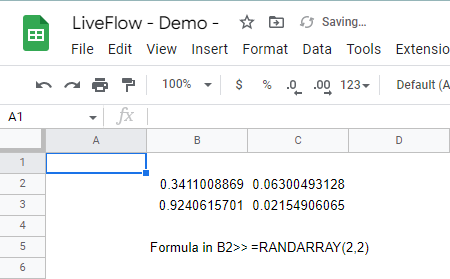# RANDARRAY Function in Google Sheets: Explained

## What is the RANDARRAY function in Google Sheets?

The RANDARRAY function in Google Sheets is a function that generates a random array of numbers between 0 and 1, which you can use to populate a range of cells in a spreadsheet with random values.

## How do I use the RANDARRAY function in Google Sheets?

Here is the syntax for the RANDARRAY function:

``=RANDARRAY([rows], [columns])``

rows: The number of rows in the array.

columns: The number of columns in the array.

Note that the two parameters are optional, so you can leave them blank. In that case, the formula shows a 1x1-sized array, a single random value.

For example, if you wanted to generate a 2x2 array of random values between 0 and 1, you could use the following formula:

``=RANDARRAY(2, 2)``

This would generate a 2x2 array of random values between 0 and 1, like this:How to generate the 2x2-sized array showing random numbers between 0 and 1 in Google Sheets

Learn how to do this step-by-step in the video below 👇

## Need help?

Our team is here to help you any time between 9am and 10pm EST.Email us at: help@liveflow.io